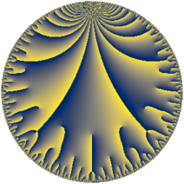# Properties

 Label 33.14.eLevel $33$ Weight $14$ Character orbit 33.e Rep. character $\chi_{33}(4,\cdot)$ Character field $\Q(\zeta_{5})$ Dimension $104$ Sturm bound $56$

# Related objects

## Defining parameters

 Level: $$N$$ $$=$$ $$33 = 3 \cdot 11$$ Weight: $$k$$ $$=$$ $$14$$ Character orbit: $$[\chi]$$ $$=$$ 33.e (of order $$5$$ and degree $$4$$) Character conductor: $$\operatorname{cond}(\chi)$$ $$=$$ $$11$$ Character field: $$\Q(\zeta_{5})$$ Sturm bound: $$56$$

## Dimensions

The following table gives the dimensions of various subspaces of $$M_{14}(33, [\chi])$$.

Total New Old
Modular forms 216 104 112
Cusp forms 200 104 96
Eisenstein series 16 0 16

## Trace form

 $$104 q + 4 q^{2} - 107220 q^{4} + 62716 q^{5} - 93312 q^{6} - 618434 q^{7} - 147428 q^{8} - 13817466 q^{9} + O(q^{10})$$ $$104 q + 4 q^{2} - 107220 q^{4} + 62716 q^{5} - 93312 q^{6} - 618434 q^{7} - 147428 q^{8} - 13817466 q^{9} + 24309448 q^{10} + 3589310 q^{11} - 23730408 q^{12} + 9535294 q^{13} - 5859830 q^{14} + 32176602 q^{15} - 669648320 q^{16} + 610397518 q^{17} - 3188646 q^{18} + 112197484 q^{19} + 1160287374 q^{20} + 1372257936 q^{21} + 2028540434 q^{22} - 5998636140 q^{23} - 3381963678 q^{24} - 2569549128 q^{25} + 9669547898 q^{26} - 11388764334 q^{28} + 6134132956 q^{29} + 10237285764 q^{30} - 4685530540 q^{31} + 15988735848 q^{32} - 6059589426 q^{33} + 63865020416 q^{34} - 72623732832 q^{35} - 56981104020 q^{36} + 139160579814 q^{37} - 1156959386 q^{38} - 59393122992 q^{39} - 229327181474 q^{40} - 131911291388 q^{41} - 29417812308 q^{42} + 510450771856 q^{43} + 264956282822 q^{44} + 33329853756 q^{45} - 648519262958 q^{46} + 142552314382 q^{47} + 482819324400 q^{48} + 108542572396 q^{49} + 85214949606 q^{50} - 263800069056 q^{51} - 1521690590618 q^{52} - 245343016054 q^{53} + 198359290368 q^{54} + 1447100453824 q^{55} + 536694949860 q^{56} - 863552731392 q^{57} + 1825280774526 q^{58} + 208343291606 q^{59} + 562558642020 q^{60} - 1256793454194 q^{61} + 508458612808 q^{62} - 328661183394 q^{63} - 3098082462864 q^{64} - 2841196991804 q^{65} + 915623784216 q^{66} + 733058038748 q^{67} + 9340513515352 q^{68} - 841127867622 q^{69} - 2499086305044 q^{70} - 9759334852284 q^{71} + 95756102262 q^{72} + 2986664977964 q^{73} + 12222409173438 q^{74} - 6089350594392 q^{75} - 2677138036736 q^{76} + 1482195769166 q^{77} + 8468819768880 q^{78} + 5939912360202 q^{79} - 8025202490062 q^{80} - 7343167948506 q^{81} - 21034958295710 q^{82} - 9123299322190 q^{83} - 20050865743428 q^{84} + 15165980627086 q^{85} + 46148120251624 q^{86} + 28726428900456 q^{87} - 12238694915072 q^{88} - 4203472457444 q^{89} - 2807460376812 q^{90} - 27210690309594 q^{91} - 31934141844130 q^{92} - 6437524379868 q^{93} - 17728422468234 q^{94} + 29869083230190 q^{95} + 27601068665502 q^{96} - 19479151080510 q^{97} + 9538842365096 q^{98} - 6966915160680 q^{99} + O(q^{100})$$

## Decomposition of $$S_{14}^{\mathrm{new}}(33, [\chi])$$ into newform subspaces

The newforms in this space have not yet been added to the LMFDB.

## Decomposition of $$S_{14}^{\mathrm{old}}(33, [\chi])$$ into lower level spaces

$$S_{14}^{\mathrm{old}}(33, [\chi]) \cong$$ $$S_{14}^{\mathrm{new}}(11, [\chi])$$$$^{\oplus 2}$$